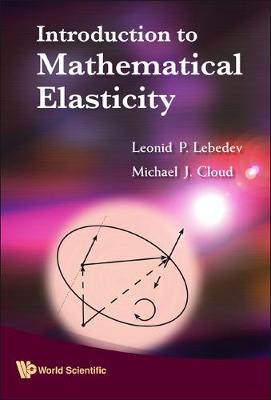•# Introduction To Mathematical Elasticity (Hardback)

(author), (author)
£104.00
Hardback 316 Pages / Published: 04/09/2009
• We can order this

Usually dispatched within 3 weeks

This book provides the general reader with an introduction to mathematical elasticity, by means of general concepts in classic mechanics, and models for elastic springs, strings, rods, beams and membranes. Functional analysis is also used to explore more general boundary value problems for three-dimensional elastic bodies, where the reader is provided, for each problem considered, a description of the deformation; the equilibrium in terms of stresses; the constitutive equation; the equilibrium equation in terms of displacements; formulation of boundary value problems; and variational principles, generalized solutions and conditions for solvability.Introduction to Mathematical Elasticity will also be of essential reference to engineers specializing in elasticity, and to mathematicians working on abstract formulations of the related boundary value problems.

Publisher: World Scientific Publishing Co Pte Ltd
ISBN: 9789814273725
Number of pages: 316
Weight: 603 g
Dimensions: 246 x 169 x 24 mm